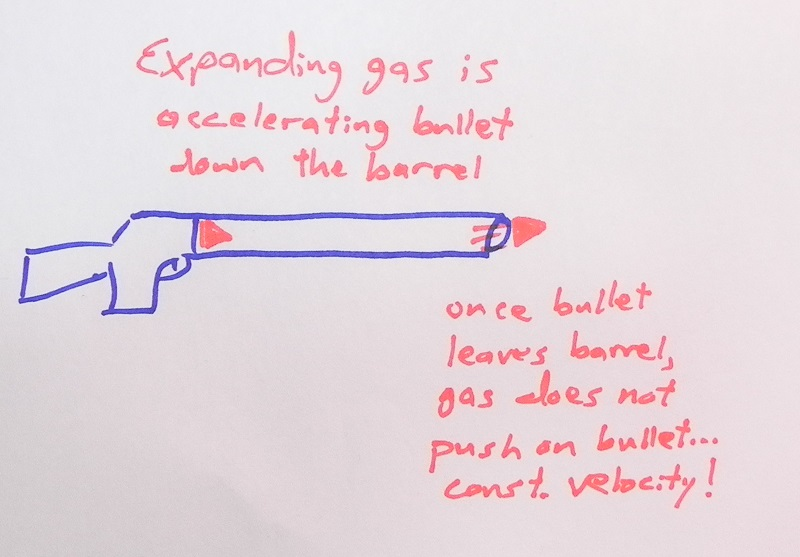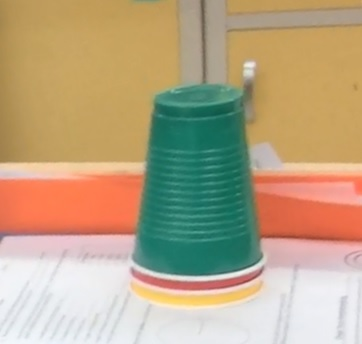Combining Newton's Second Law and Kinematics

5 teachers like this lesson
Print Lesson

Objective

Students solve a variety of problems with Newton's Second Law and the equations of motion.

Big Idea

Kinematics equations and Newton's 2nd Law allow us to better understand the world around us and to make predictions about the motion of an object.

Context

In a past lesson, students applied Newton's 2nd Law to solve sample, real-world problems. Students continue to solve problems using Newton's 2nd Law that also incorporate the kinematics equations. It is important to inform students that the topics we study are not separate and isolated from one another, but are in fact connected and need to be used together to truly understand our world.

This lesson involves Performance Standard HS-PS2-1, the mathematical relationship between the net force acting on an object and its acceleration. In order to successfully complete this lesson, students use Science Practice 5: Using mathematics and computational thinking and Constructing explanations (for science). Math Practice 2: Reason abstractly and quantitatively, is also part of this lesson, as students problem-solve real-life situations using the kinematic equations and Newton's 2nd Law.

Lecture on Advanced Concepts

10 minutes

I give this short lecture to model effective problem solving for the practice set given later in the lesson. I choose to lecture here because it serves as an efficient way to pass on information to students. Students take notes projected on the board with Newtons 2nd Law and Kinematics.

The first slide is the objective of today's class period; it is important to let inform the students of what they will do during the class. The second and third slides are reminders on the concept of weight and F=mg. Students have been exposed to this concept but this is the first time they are putting it in their notebook.

The remainder of the slides is problem solving that combines Newton's Second Law and the kinematic equations in a single problem. I display the problem and give students 2-3 minutes to write down the problem and work through a solution. Then I solve the problem using the document camera, stepping the students through my thought process on how I tackle the problem.As a part of my solution to the last problem of a bullet being fired, I include a diagram of the gun so I can point out that the bullet accelerates only while it travels inside the barrel. Once it leaves the barrel, we can consider the velocity constant. I inform students that visual representations are extremely useful in problem solving and that being able to picture a situation is as important as applying any equations. Graphs are also useful and so I decide to draw the three graphs of motion (position versus time, velocity versus time and acceleration versus time) and challenge students to draw these for the bullet being fired from the riffle in the last sample problem. I encourage students to discuss their solutions with their neighbors and I walk the classroom giving support where needed. After 2-3 minutes, I reveal the answers, again sharing my thought process as I complete the exercise.

Students Practice Problems

25 minutes

This worksheet, Newtons 2nd Law and Kinematics Practice, has a variety of multiple-choice problems and word problems. The questions cover material given since the beginning of the unit to remind students of what they are expected to know and be able to do. I hand out the worksheet and inform the students that they have 25 minutes to complete this sheet and I collect it at the end of the period. This practice helps students develop expertise in the content and makes them move effective problem solvers. For the more advanced students, I include a challenging bonus problem at the end of the worksheet.While students are working on the sheet, I circulate the classroom and give support where needed. In order to better serve the students who need my help, I have devised a three cup system that alerts me to who needs help. My students sit at tables in groups of four. At each table are three cups, a red one, a yellow one and a red one (pictured to the right). Depending on how students are doing in the assignment, they will put one of the colored cups on top.

Green Cup: default cup, everything is going well, we don't need help.

Yellow Cup: We are struggling with a concept, we need you to affirm that we are heading in the right direction.

Red Cup: Help! We are totally stuck and can't move forward.

As students work through the assignment, I scan the room. Red cups get priority and then yellow cups. The cups are a permanent fixture at the tables and are used when ever students are engaged in student-centered activities (worksheets, laboratories, inquiries, etc). If I look up and see mostly green cups, then I know things are proceeding well. However, if mostly red cups are displayed, then this tells me that I have to stop the independent work and reteach teach the concepts.

Students have 25 minutes to work through these practice problems. Then, to finish the class period, we review these practice problems so that students get immediate feedback as they self-assess their understanding.

Correcting Newton's 2nd Law Practice Problems

15 minutes

I use my document camera to display the solutions. I display one half of the sheet front and give students an opportunity to fix any errors they have and ask questions about something that confuses them. It is important for students to be able to think about their own understanding and this self correction exercise helps them to reflect on how well they know the concepts. I also inform the students that they should make sure they understand each of the solutions as these are the types of questions that will be on the quiz happening in a few days time.

On the student work sample, we see fairly typical solutions. On the first problem, the student listed the variables but then made a mistake copying down the kinematic equation. I instruct my students to always write down the equations they use because this makes finding mistakes much easier. Also fairly typical is the this student did not attempt the bonus problem. Usually only 1 or 2 students are able to successfully work through this problem as it has multiple steps and multiple accelerations, which is confusing to most students.